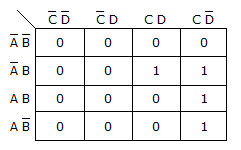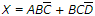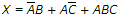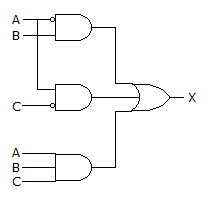# Digital Electronics - Combinational Logic Circuits

### Exercise :: Combinational Logic Circuits - True or False

31.

Truth tables are great for listing all possible combinations of independent variables.

 A. True B. False

Explanation:

No answer description available for this question. Let us discuss.

32.

The K-map in the figure below shows the correct implementation of the expression X = ACD + AB(CD + BC).A. True B. False

Explanation:

No answer description available for this question. Let us discuss.

33.

A square in the top row of a K-map is considered to be adjacent to its corresponding square in the bottom row.

 A. True B. False

Explanation:

No answer description available for this question. Let us discuss.

34.is in the form of a sum-of-products expression.

 A. True B. False

Explanation:

No answer description available for this question. Let us discuss.

35.

The circuit given below implements the equation,.A. True B. False